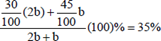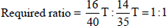### CAT 2017 – Slot 1 – Quantitative Ability – If a seller gives a discount of 15% on retail price, she still makes a profit of 2%

Q. 1: If a seller gives a discount of 15% on retail price, she still makes a profit of 2%. Which of the following ensures that she makes a profit of 20%?
A) Give a discount of 5% on retail price
B) Give a discount of 2% on retail price
C) Increase the retail price by 2%
D) Sell at retail price

Let Retail Price = MP

Cost Price = CP

When a discount of 15% is given,

[(0.85CP – CP)/CP] *100 = 2

So, 85 * MP = 102 * CP

Let us take SP = xMP

So, [(xMP-CP)/CP] * 100 = 20.

On calculating, we get x = 100

So, we should sell at Retail Price to get a profit of 20%.

##### Q. 2: A man travels by a motor boat down a river to his office and back. With the speed of the river unchanged, if he doubles the speed of his motor boat, then his total travel time gets reduced by 75%. The ratio of the original speed of the motor boat to the speed of the river isA) √6 / √2B) √7 / 2C) 2√5 / 3D) 3: 2

Total travel time gets reduced by 75%. So, total travel time becomes 1/4th of the original.

So, s’(New speed) = 4s(Original speed)

Speed of boat : Speed of river = x:1

When the speed of boat is normal

Speed Upstream = x-1

Speed Downstream = x+1

Here, the distance is constant. So, average speed can be found out by the harmonic mean of two speeds = 2ab/ (a+b)

Here, average speed will be 2(x^2-1)/2x = (x^2-1)/x

When the speed of boat is doubled

Speed upstream = 2x-1

Speed downstream = 2x+1

Here, the distance is constant. So, average speed can be found out by the harmonic mean of two speeds = 2ab/(a+b)

Here, average speed will be 2(4x^2-1)/4x = (4x^2-1)/2x

Now, we know that

New speed = 4*Original speed

So,

(4x^2-1)/2x = 4(x^2-1)/x

4x^2 – 1 = 8x^2 – 8

4x^2 = 7

x = √7/2

Ratio = x:1 = √7/2

Option (B)

Q. 3: Suppose, C1, C2, C3, C4, and C5 are five companies. The profits made by C1, C2, and C3 are in the ratio 9 : 10 : 8 while the profits made by C2, C4, and C5 are in the ratio 18 : 19 : 20. If C5 has made a profit of Rs 19 crore more than C1, then the total profit (in Rs) made by all five companies is
A) 438 crore
B) 435 crore
C) 348 crore
D) 345 crore

Profit of C1 = 9x

Profit of C2 = 10x = 18y — (1)

Profit of C3 = 8x

Profit of C4 = 19y

Profit of C1 = 20y

Taking eq(1), we get x = 9y/5

20y = 19 + 9x

Putting value of x and y in the above eq we get, x=9 and y=5

So, Total profit = 9x +10x + 8x + 19y + 20y = 438 crore

Option (A)

Q. 4: The number of girls appearing for an admission test is twice the number of boys. If 30% of the girls and 45% of the boys get admission, the percentage of candidates who do not get admission is
A) 35
B) 50
C) 60
D) 65

Explanation:- Let the number of boys appearing for the admission test be b. Percentage of candidates who get admission =65% of the candidates do not get admission.

Q. 5: A stall sells popcorn and chips in packets of three sizes: large, super, and jumbo. The numbers of large, super, and jumbo   packets in its stock are in the ratio 7 : 17 : 16 for popcorn and 6 : 15 : 14 for chips. If the total number of popcorn packets in its stock is the same as that of chips packets, then the numbers of jumbo popcorn packets and jumbo chips packets are in the ratio
A) 1: 1
B) 8: 7
C) 4: 3
D) 6: 5

Explanation:- Let the total number of popcorn packets in stock be T. Total number of chips packets in stock = T##### Checkout Other Questions of CAT 2017 Slot 1 Paper:

Verbal Ability :              |   Q.01- Q.06  |  Q.07- Q.12  |  Q.13- Q.18  |  Q.19- Q.21  |  Q.22- Q.24  |  Q.25- Q.29  |  Q.30 – Q.34  |

Logical Reasoning :    |   Q.29 – Q.32  |

Quantitative Aptitude: |